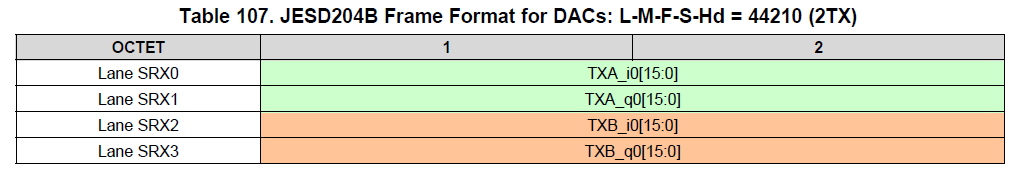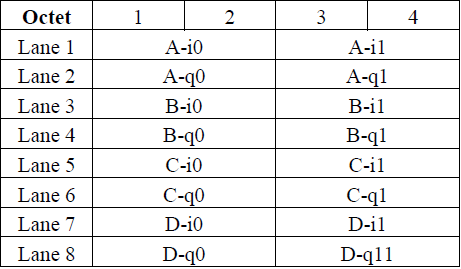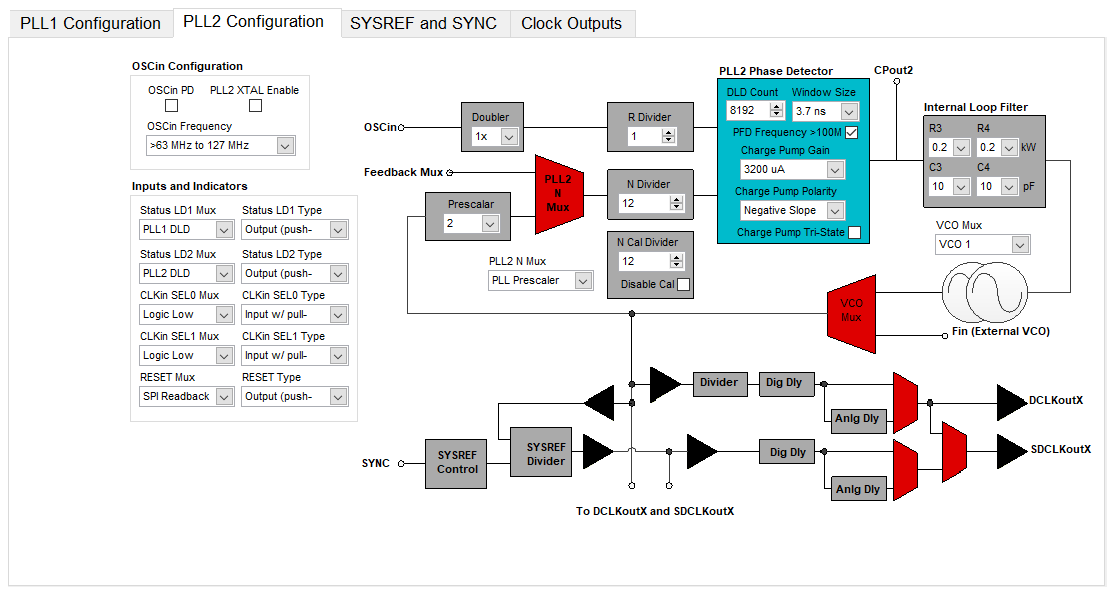• Resolved

# AFE7444: What are the actual definitions of L-M-S-F for KCU105 AFE74xx XCVR 2x44210 JESD Reference Design

Part Number: AFE7444

Hi everyone,

I am currently trying to understand the reference design KCU105 AFE74xx XCVR 2x44210 JESD. I would like to understand the JESD mode 2x44210 as mentioned in the reference design user guide. I looked at different documents regarding what the L-M-S-F meant and got more confused than able to understand them clearly. I referred to the following sources and tried to collaborate the understanding with the FPGA block diagram provided in the reference design.

The definitions for L-M-F-S varied in both the sources and I could not make sense anymore.

I wanted the SerDes line rate 7.3728 Gbps and hence looked at the mode corresponding to that in 'AFE74xxEVM User's Guide' and found the following table

Then from the following table

Can someone clarify what the following terms are, in the FPGA block diagram and also with other illustrations if possible

1) Converters

2) Lanes

3) Number of frames for a particular mode (2x44210 here)

Basically the whole L-M-F-S part

-Chandrasekhar DVS

• Hi Chandrasekhar,

Frame format of LMFS: 44210 is shown in full datasheet Table 107:Here LMFS is specified as 44210 for 2 Tx or 2 Rx analog (physical) channels. For all four channels, it's specified as 2x44210. Each Tx is configured with a complex DUC and Rx with a complex DDC. So each channel has an I/Q pair (I  and Q channels). So number of converters (for JESD) is 4 per 2 physical channels.

L (number of lanes) is 4 per 2Tx (8 per all 4Tx)

M (number of converters) is 4 (AI, AQ, BI and BQ) per 2Tx (8 for all 4Tx i.e AI, AQ, BI, BQ, CI, CQ, DI and DQ)

F (number of octets per frame) is 2 (one 16-bit sample per lane per frame. So 2 octets)

S (number of samples per frame) is 1.

This LMFS mode can be easily understood as, each I or Q channels data is received/ transmitted on one JESD lane. Total 8 converters (4 I/Q pairs) data is received/ transmitted on 8 SERDES lanes..

Regards,

Vijay

• In reply to Vijayendra Varma Siddamsetty:

Thanks Vijay!

Also, there is no specification for the number of frames (per lane? or per converter?). In the reference design,  the sample format for JESD Mode 2x44210 was given asSo, I would like to know a few more things,

1) How the frames are defined for the reference design (As in 'frames per lane' or 'frames per converter'). I would like to know about that.

2) In this case where probably multiple frames are used,  LMFC (Local Multi-Frame Clock) goes from low to high at the start of every multi-frame? (Here, every two frames per lane).

If you or someone could also clarify this, it would be helpful.

-Chandrasekhar DVS

• In reply to Chandrasekhar Dhulipala:

Hi Chandrasekhar,

1) How the frames are defined for the reference design (As in 'frames per lane' or 'frames per converter'). I would like to know about that.

The sample format shown in the reference design shows two frames.

There are 2 octets per lane per frame. (as F = 2 in LMFS = 4421). Each frame has one sample from each converter  (as S = 1 in LMFS = 4421).

One frame is 2 octets from each lanes as shown in the Table 107 in the datasheet.

2) In this case where probably multiple frames are used,  LMFC (Local Multi-Frame Clock) goes from low to high at the start of every multi-frame? (Here, every two frames per lane).

In GUI, by default, number of frames per multi-frame (K) is 32. LMFC goes from low to high at start of every multi-frame which is 32 frames wide.

Regards,

Vijay

• In reply to Vijayendra Varma Siddamsetty:

Hi Vijay,

I would also like to understand the different clocks used in the reference design.

 1. Refclk (given to the AFE74xx EVM) The typical value of Refclk is 368.64 MHz 2. cpll_refclk and qpll0_refclk (to the FPGA) The cpll_refclk and qpll0_refclk are set to 184.32 MHz when I set the data rate to be 7.3728 Gbps (which is equal to serial data rate/40) 3. LMFC (Local multiframe clock) No idea what this LMFC clock frequency is and how this clock frequency is decided. 4. SYSREF clock on AFE74xx EVM side SYSREF pulse should be source synchronous to device clock. Does that mean the SYSREF clock on AFE74xx EVM be equal to the reference frequency? If not, how do I calculate the SYSREF clock frequency? 5. Clock to DAC For the 44210 (2Tx/Rx) mode, the interpolation factor for DAC is 24x. Hence the clock is 24 x 368.64 MHz = 8847.36 GHz 6. Clock to ADC The clock for ADC should be DAC clock /2 or /3 or /4 and should be < 3 GHz. Hence for /3 , the clock is 2949.12 GHz

I would like to know if the above interpreted information is accurate for the mode in question and how to calculate LMFC.

Also you spoke about frame clock frequency right? How do I calculate that?

Can you please guide me here?

-Chandrasekhar DVS

• In reply to Chandrasekhar Dhulipala:

Hi Chandrasekhar,

Your interpretation and frequencies of Ref clock to AFE, Ref clock to FPGA, DAC and ADC sampling clocks are correct. Please note that ref clock to AFE and FPGA are provided by LMK04828 on EVM. DAC clock is generated by internal PLL of the AFE. Divided-by-3 clock of internal PLL output is used as ADC clock.

In this mode, Tx input data rate before interpolation and Rx output data rate after decimation are both 368.64 MSPS. (Note that this need not be equal to frequency of ref clock to AFE which is also 368.64 MHz in this case coincidentally)

As S=1, one frame has one output/ input sample from each DAC or ADC (before interpolation or after decimation). So here frame clock frequency is equal to output/ input data rate which is 368.64 MHz.

Number of frames per multi-frame is 32. So, Multi-frame clock frequency is (frame clock frequency)/ 32 = 368.64/32 = 11.52 MHz.

SYSREF frequency is required to be a subharmonic of the LMFC frequency. By default it's chosen to be 0.96 MHz by EVM GUI. More details on SYSREF frequency calculation can be found in section 7.3.4.4.2 of dataheet.

Regards,

Vijay

• In reply to Vijayendra Varma Siddamsetty:

Hi Vijay,

Vijayendra Varma Siddamsetty
Number of frames per multi-frame is 32. So, Multi-frame clock frequency is (frame clock frequency)/ 32 = 368.64/32 = 11.52 MHz.

So when I navigated to section 7.3.4.4.2 of the datasheet, I saw a formula to calculate LMFC which is given as

LMFC = Lane Rate /40/F/K  (assuming that the LMFS is a typo in the document as it does not make sense when referring to the example in the same page)

F: Number of Octets per frame

K: Number of frames per lane

Lane rate: SERDES lane rate

For the mode in the reference design LMFS - 44210, the Lane rate is 7.3728 Gbps, K=32, and F=2. Substituting the values, I get a different value (2.88 MHz). Now why is this different from what you mentioned?

Could you please clarify this section?

Vijayendra Varma Siddamsetty
SYSREF frequency is required to be a subharmonic of the LMFC frequency. By default it's chosen to be 0.96 MHz by EVM GUI

Can you point out how you figured this out? Because when I look at the different tabs in EVM GUI, there is no such obvious value I see anywhere and I could not interpret from anywhere.

Below are the PLL2 and SYSREF and SYNC tabs in the EVM GUI. After applying all the values. Can you point out the relevant sections in them?-Chandrasekhar DVS

• In reply to Chandrasekhar Dhulipala:

Hi Chandrasekhar,

Generally, LMFC frequency is equal to (Lane rate/ 10/ F/ K) for 8 bit/10 bit data encoding. In the FPGA, LMFC is 11.52MHz. For AFE7444, due to internal digital clocking architecture, there's an additional factor of 4 in the denominator. So your calculation is right LMFC is 2.88 MHz and SYSREF has to be a sub harmonic of 2.88 MHz. 0.96 MHz still satisfies this condition.

SYSREF frequency from LMK is equal to (PLL2 VCO output: 2949.12)/(SYSREF divider). In the screen-shot you sent, SYSREF divider value is 256. But after EVM is programmed this should be changed to 3072. Then SYSREF = 2949.12/3072 = 0.96 MHz.

Regards,

Vijay

• In reply to Vijayendra Varma Siddamsetty:

Hi Vijay,

Vijayendra Varma Siddamsetty
In the screen-shot you sent, SYSREF divider value is 256. But after EVM is programmed this should be changed to 3072. Then SYSREF = 2949.12/3072 = 0.96 MHz.

Now what do you mean by divider value changes from 256 to 3072 after the EVM is programmed? After applying all the configuration settings, I saw the SYSREF divider value go from 3072 to 256.

Can you please explain what you meant and what relevance does the value of SYSREF divider hold, once the EVM  is programmed?

-Chandrasekhar DVS

• In reply to Chandrasekhar Dhulipala:

Hi Chandrasekhar,

I verified on EVM and after programming SYSREF divider value is 256. This means SYSREF is set to 11.52 MHz. When I said 3072, I didn't have EVM setup and was running GUI in simulation mode. Please ignore the value of 3072. Sorry for the confusion.

This doesn't satisfy the requirement that SYSREF should be a sub-harmonic of LMFC. However it is proven to be working on the EVM. So it's acceptable for the evaluation platform. Please make sure this requirement is satisfied in the your system (SYSREF divider of 3072 can be used).

Regards,

Vijay

• In reply to Vijayendra Varma Siddamsetty:

Hi Vijay,

We will be working with the EVM initially. However, we would need to do a little R&D with the AFE7444 chip for our application. In such a case, it would be helpful to understand the basics of the reference design. When you say that SYSREF divider value of 256 does not satisfy the sub-harmonic condition, why does the GUI setup that value? I ask this because at a later point where we might have to decide on changing the configuration (The operating mode for JESD interface, the reference clock, for AFE7444 chip) we would like to predict the dependent parameters.

Could you suggest how the dependencies can be predicted in a consistent way?